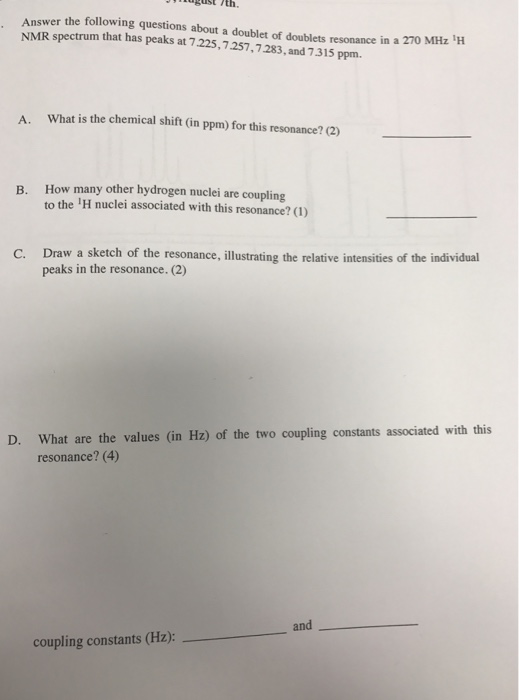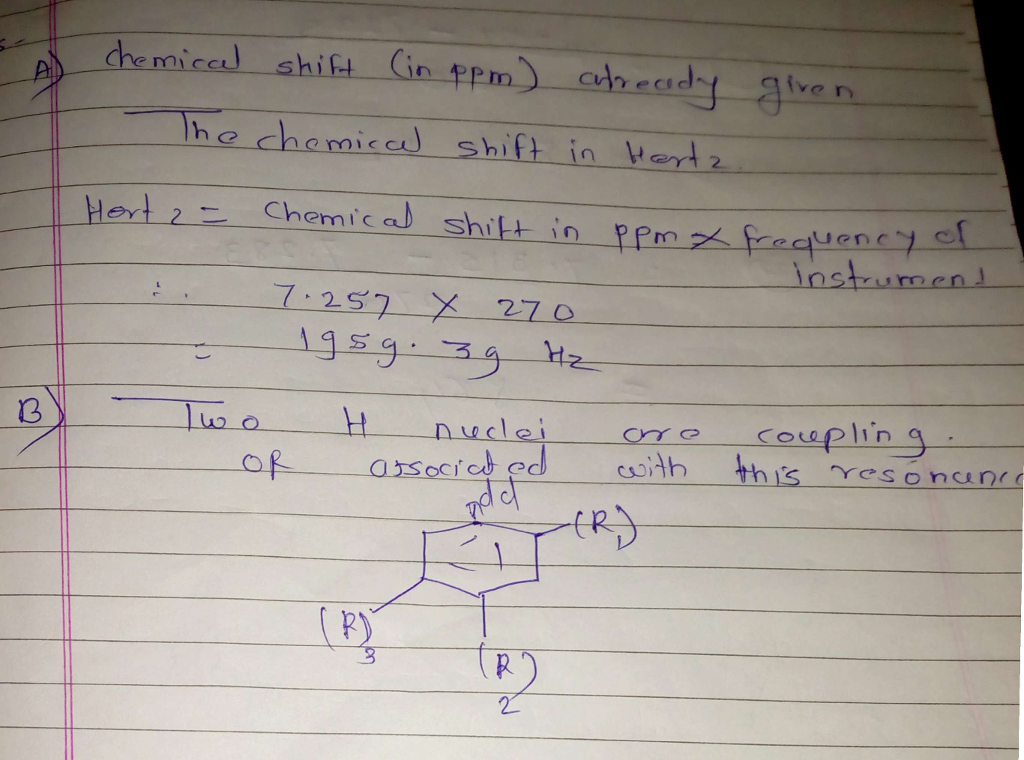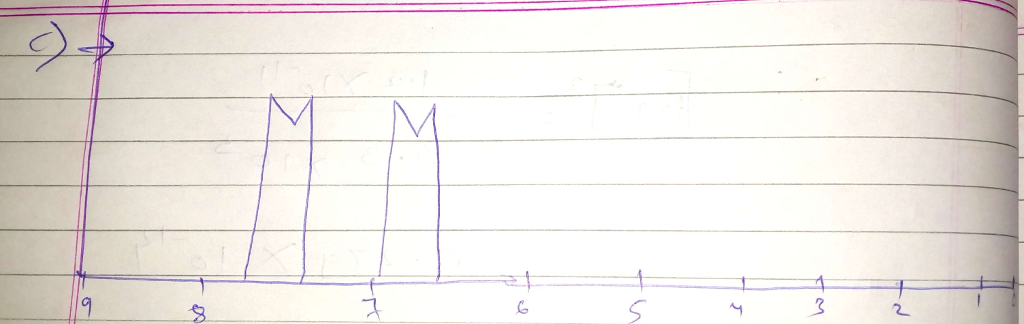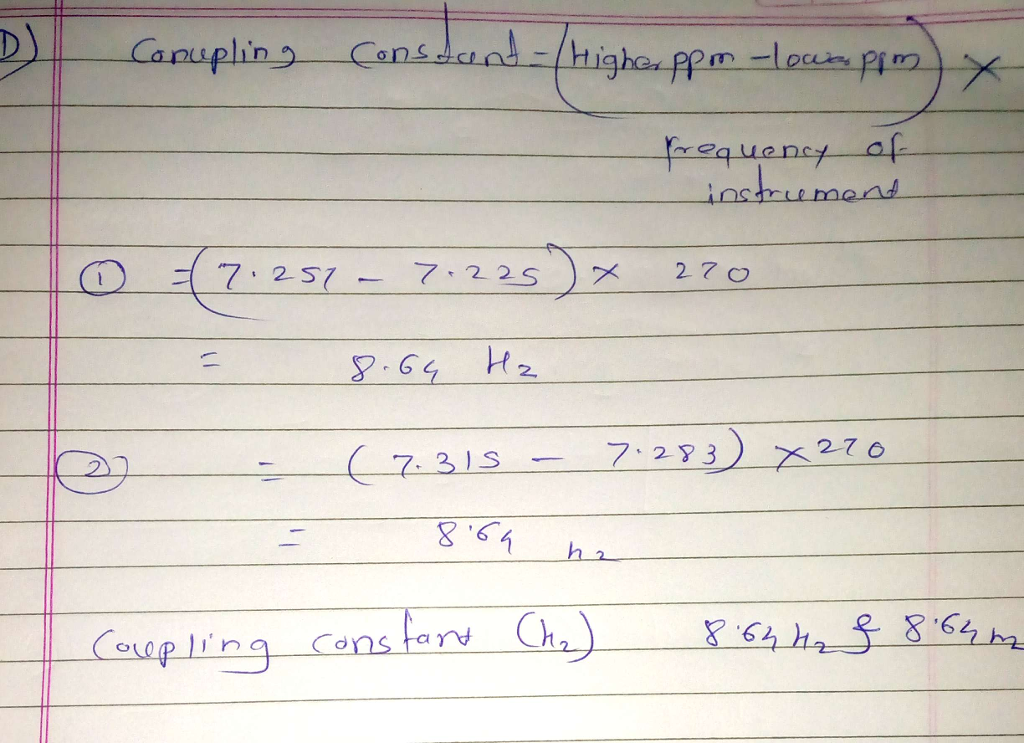# Question & Answer: Answer the following questions about a doublet of doublets resonance in a 270 MHz ^1 H NMR…..Answer the following questions about a doublet of doublets resonance in a 270 MHz ^1 H NMR spectrum that has peaks at 7.225, 7.257, 7.283, and 7.315 ppm. A. What is the chemical shift (in ppm) for this resonance? __________ B. How many other hydrogen nuclei are coupling to the ^1 H nuclei associated with this resonance? __________ C. Draw a sketch of the resonance, illustrating the relative intensities of the individual peaks in the resonance. D. What are the values (in Hz) of the two coupling constants associated with this resonance? coupling constants (Hz): ___________ and _________